### Home > CAAC > Chapter 6 > Lesson 6.2.2 > Problem6-53

6-53.

Examine the balanced scales in Figures 1 and 2 shown below. Figure 1 shows that two candies balance three dice. Figure 2 shows that one rubber ball balances two jacks. Homework Help ✎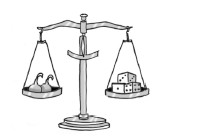Figure 1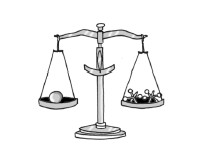Figure 2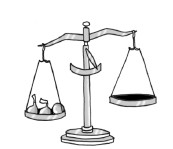Figure 3

Determine what could be placed on the right side of the scale in Figure 3 to balance with the left side. Justify your solution in complete sentences.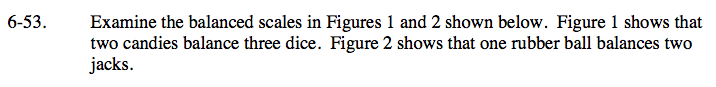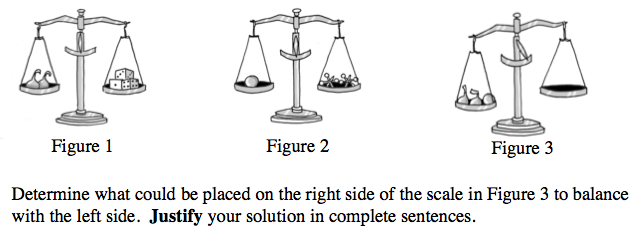2 candies = 3 dice

1 ball = 2 jacks

Therefore, 2 candies + 1 ball = ?

3 dice + 2 jacks